# High School Math : Hexagons

## Example Questions

← Previous 1

### Example Question #1 : How To Find The Perimeter Of A Hexagon

What is the perimeter of a regular hexagon with a side length of 12?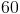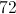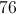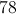Explanation:

To find the perimeter of a regular hexagon you must first know the number of sides in a hexagon which is 6.

When you know the number of sides of a regular polygon to find the perimeter you must multiply the side length by the number of sides.

In this case it is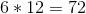The answer for the perimeter is.

### Example Question #2 : How To Find The Perimeter Of A Hexagon

How many degrees is the interior angle of any regular hexagon?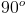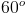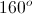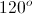Explanation: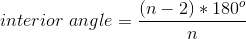To find the angle of any regular polygon, you find the number of sides,. In this example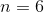.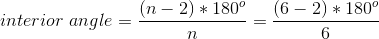You then subtractfrom the number of sides, yielding.

Takeand multiply it by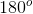to yield the total number of degrees in the regular hexagon.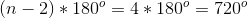Then, to find one individual angle, we divide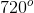by the total number of angles,.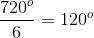The answer is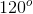.

### Example Question #3 : How To Find The Perimeter Of A Hexagon

What is the perimeter of a hexagon with a side length of?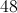Explanation:

To find the perimeter of a regular polygon, we take the length of each side,, and multiply it by the number of sides,.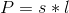In a hexagon the number of sides is, and in this example the side length is.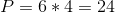The perimeter is.

### Example Question #4 : How To Find The Perimeter Of A Hexagon

How many lines of symmetry can be found in a regular hexagon?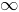Explanation:

The number of lines of symmetry through a regular polygon is equal to the number of sides.

A hexagon has lines of symmetry through each vertex, giving three lines of symmetry that each connect two opposite vertices. The other three lines pass through the midpoints of opposite sides of the hexagon.

### Example Question #5 : How To Find The Perimeter Of A Hexagon

What is the perimeter of a regular hexagon with a side length of?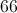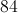Explanation:

To find the perimeter of a regular hexagon you must first know the number of sides in a hexagon which is.

When you know the number of sides of a regular polygon to find the perimeter you must multiply the side length by the number of sides.

In this case it isThe answer for the perimeter is.

### Example Question #6 : How To Find The Perimeter Of A Hexagon

This figure is a regular hexagon with one side measuring 6 cm.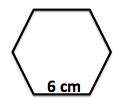What is the perimeter of the regular hexagon in centimeters?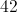Explanation:

The perimeter of a regular hexagon is just the sum of all 6 sides.  Because it's a regular hexagon, the perimemter is just six times one side (6 cm) or 36 cm.

### Example Question #1 : How To Find The Length Of The Diagonal Of A Hexagon

What is the length of a diagonal of a regular hexagon with side length?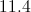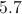Explanation:

Regular hexagons are comprised of six equilateral triangles; in our question, these triangles each have side length 4 (see diagram).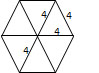The length of a diagonal is equal to two times the length of the side. In this instance, the answer is 8.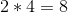### Example Question #3 : Hexagons

How many diagonals are there in a regular hexagon?Explanation:

A diagonal is a line segment joining two non-adjacent vertices of a polygon.  A regular hexagon has six sides and six vertices.  One vertex has three diagonals, so a hexagon would have three diagonals times six vertices, or 18 diagonals.  Divide this number by 2 to account for duplicate diagonals between two vertices. The formula for the number of vertices in a polygon is: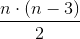where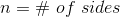.

### Example Question #4 : Hexagons

How many diagonals are there in a regular hexagon?

9

18

10

6

3

9

Explanation:

A diagonal connects two non-consecutive vertices of a polygon.  A hexagon has six sides.  There are 3 diagonals from a single vertex, and there are 6 vertices on a hexagon, which suggests there would be 18 diagonals in a hexagon.  However, we must divide by two as half of the diagonals are common to the same vertices. Thus there are 9 unique diagonals in a hexagon. The formula for the number of diagonals of a polygon is:where n = the number of sides in the polygon.

Thus a pentagon thas 5 diagonals.  An octagon has 20 diagonals.

### Example Question #7 : Hexagons